#INFOSWMM

Weir Background Equations for #SWMM5 and #InfoSWMM

Weir Background Equations for #SWMM5 and #InfoSWMM

Introduction: H2OCalc is a hydrology and hydraulics calculator sold by Innovyze that has many of the equations and calculation methods used in InfoSWMM, ICM and SWMM5. This is the H2OCalc information for the Weir Equations.

Discharge in channels and small streams can be conveniently measured by using a weir. Weirs can be categorized in to two: sharp crested and broad crested.

Sharp-Crested Weir

A sharp-crested weir is a vertical plate placed in a channel that forces the liquid to flow through an opening to measure the flow rate. The type of the weir is characterized by the shape of opening.

Rectangular Sharp-Crested Weir

A vertical thin plate with a straight top edge is referred to as rectangular weir since the cross section of the flow over the weir is rectangular (see the following figure).The discharge equation for a rectangular weir is given as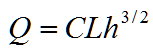where Q = discharge over the weir (m3/s, ft3/s)

L = weir length (m, ft)

C = weir coefficienttypically given as 1.84 in SI, 3.33 in English.

Flow through the weir may not span the entire width of the channel (L) due to end contractions. Experiments have indicated that the reduction in length is approximately equal to 0.1nh, where n is the number of end contractions (e.g., could be 2 in the contracted rectangular weir), and h is head over the crest of the weir as defined above. Therefore, the formula for contracted weir (one with flow contraction due to end walls) is given asMultiple-Step Sharp Crested Rectangular Weir

A multiple step weir is a rectangular weir with stepwise increase in length along the weir height. It helps to maintain low velocity across the weir during low flows and may be ecologically friendly as it allows fish freely pass across the weir.The discharge equation for multi-step weirs is given as: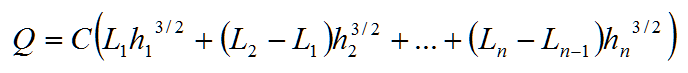where Q = discharge over weir (m3/s, ft3/s)

hi = head over the crest of the weir at step i (m, ft)

Li = length of the weir at step i (m, ft)

C = the flow coefficient (1.86 in SI, 3.367 in English)

Cipolletti Sharp-Crested Weir

The Cipolletti (or trapezoidal) weir has side slopes of 4 vertical to 1 horizontal ratio as shown in the figure below. The discharge equation for a Cipolletti weir is given as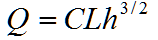where Q = discharge over weir (m3/s, ft3/s)

L = weir bottom length (m, ft)

C = the flow coefficient (1.86 in SI, 3.367 in English)Notice that L is measured along the bottom of the weir (called the crest), not along the water surface.

V-Notch Sharp-Crested Weir

With low flow rate, it is common to use a V-Notch weir (shown below).The discharge equation for a V-Notch weir is given aswhere Q = discharge over weir (m3/s, ft3/s)

θ = angle of notch (degree)

C = the flow coefficient that typically range between 0.58 and 0.62.

The most commonly used value of the notch angle θ is 90o; for this case (i.e., θ is 90o), C is found to be around 0.585.

Submerged Sharp-Crested Weir

The weir equations discussed above assume that the weir is free flowing. However, if the tailwater rises high enough, the weir will be submerged and the weir flow-carrying capacity will be reduced. Therefore, the discharge can be adjusted for submergence using the following equation: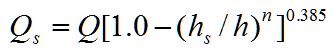where Qs = discharge over a submerged weir (m3/s, ft3/s)

Q = discharge computed using weir equations (m3/s, ft3/s)

hs = tailwater depth above the weir crest (m, ft)

h = head upstream of the weir (m, ft)

n = exponent, 1.5 for rectangular and Cipolletti weirs, 2.5 for a triangular weir.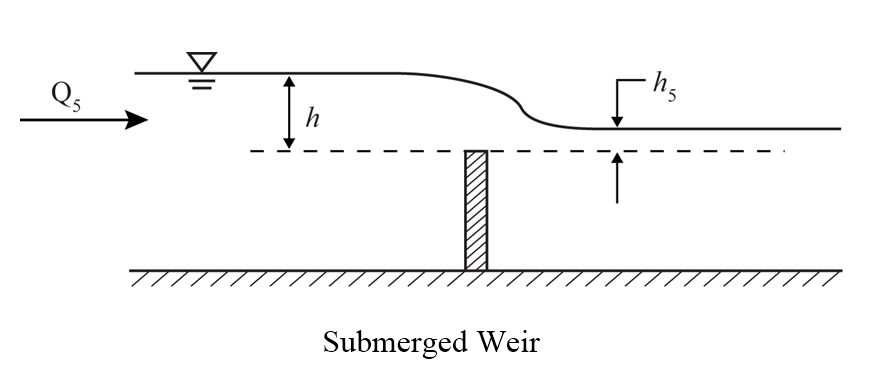If the weir is long in the direction of flow so that the flow leaves the weir in essentially a horizontal direction, the weir is a broad-crested weir.The discharge equation for a broad crested weir is given aswhere Q = discharge over weir (m3/s, ft3/s)

L = crest length (m, ft)

C = the flow coefficient that typically range between 2.4 and 3.087.

The flow coefficient C can be obtained from the following figure. Depending on the shape of the weir and head on the weir, the C value may range from 2.4 to 3.1.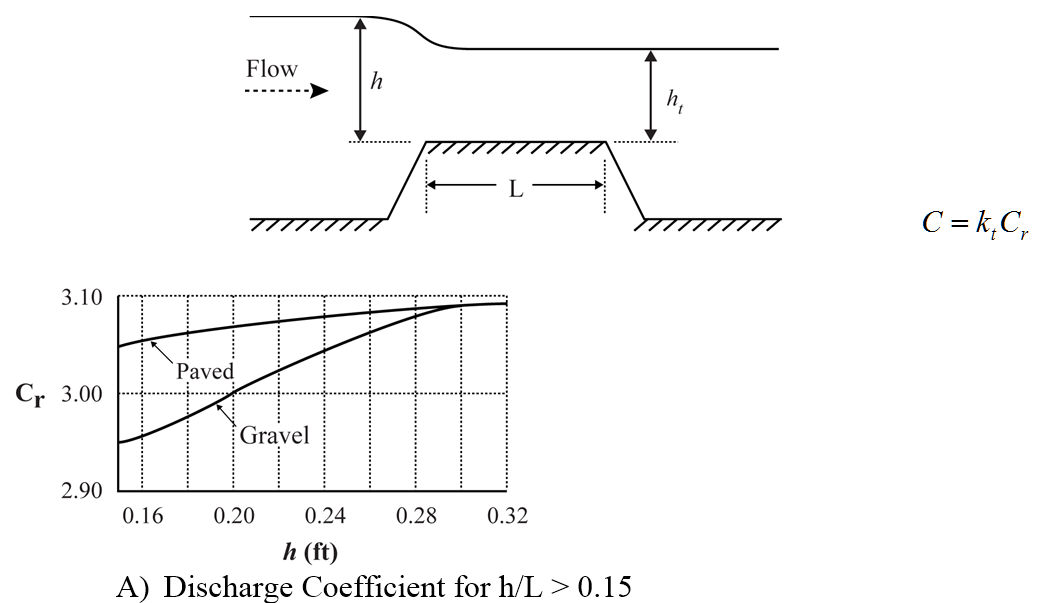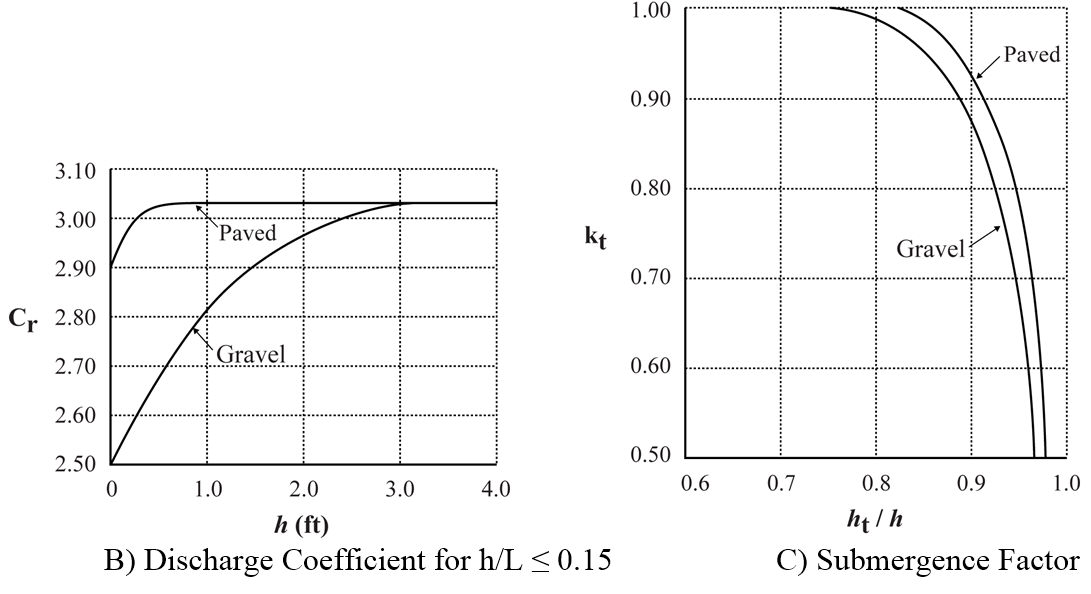Generic Weir

Any other type of weirs can be modeled as generic weir using the following equation.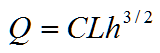where Q = discharge over weir (m3/s, ft3/s)

h = head above weir crest (m, ft)

L = crest length (m, ft)

C = weir coefficient

The weir coefficient value depends on the weir type, and is the function of the head above the weir crest.

Categories: #INFOSWMM, InfoSWMM, Innovyze, SWMM5

1.Fred Ernst says: